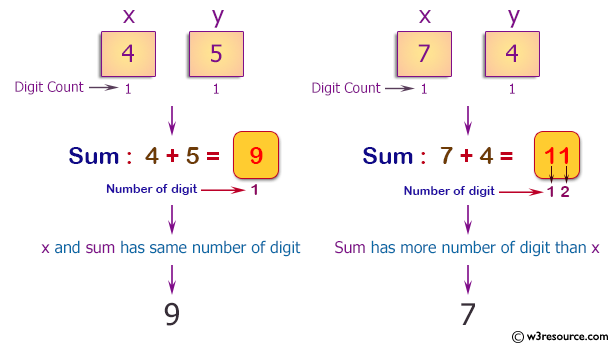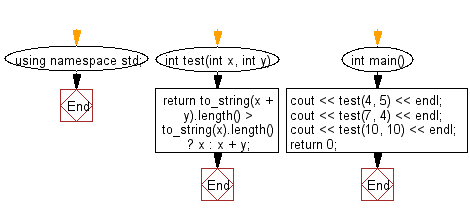﻿ C++ : Sum x and y if the sum has same number of digits as x# C++ Exercises: Compute the sum of two given non-negative integers x and y as long as the sum has the same number of digits as x

## C++ Basic Algorithm: Exercise-51 with Solution

Write a C++ program to compute the sum of two given non-negative integers x and y as long as the sum has the same number of digits as x. If the sum has more digits than x then return x without y.

Sample Solution:

C++ Code :

``````#include <iostream>
#include<string>

using namespace std;

int test(int x, int y)
{
return to_string(x + y).length() > to_string(x).length() ? x : x + y;
}

int main()
{
cout << test(4, 5) << endl;
cout << test(7, 4) << endl;
cout << test(10, 10) << endl;
return 0;
}
``````

Sample Output:

```9
7
20
```

Pictorial Presentation:Flowchart:C++ Code Editor:

Contribute your code and comments through Disqus.

What is the difficulty level of this exercise?

﻿